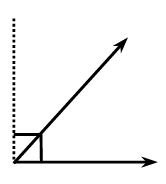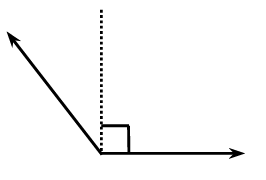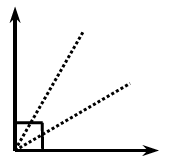### Home > ACC7 > Chapter 8 Unit 5 > Lesson CC2: 8.3.4 > Problem8-98

8-98.

You learned in an earlier lesson how to classify an angle by comparing it to a $90º$ right angle. Find the measure of each angle below and classify it according to its measure.

1. What is the measure of half of a right angle?

Remember that a right angle is $90°$.
Thus, to find the measure of half a right angle, we must divide $90°$ by $2$.

$45°$1. If the angle at right is made with a right angle added to half of a right angle, what is its measure?

In part (a), you found the measure of half a right angle.
Now you must add your answer from part (a) to $90°$ to find the measure of a right angle added to half a right angle.1. Suppose a right angle is divided into three equal angles as shown at right. What is the measure of each angle?

Similar to part (a), you must divide $90°$ by $3$ to find the measure of a third of a right angle.